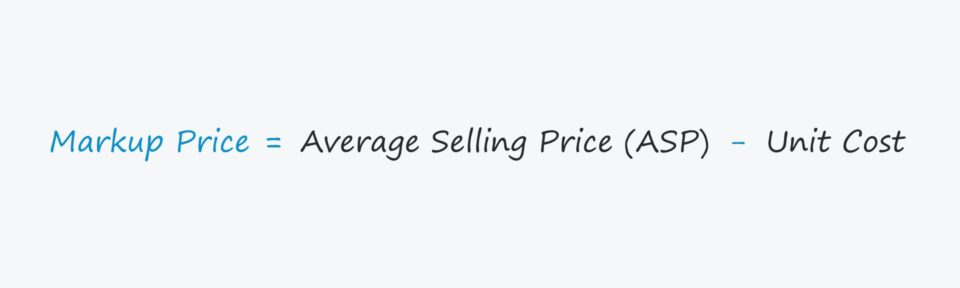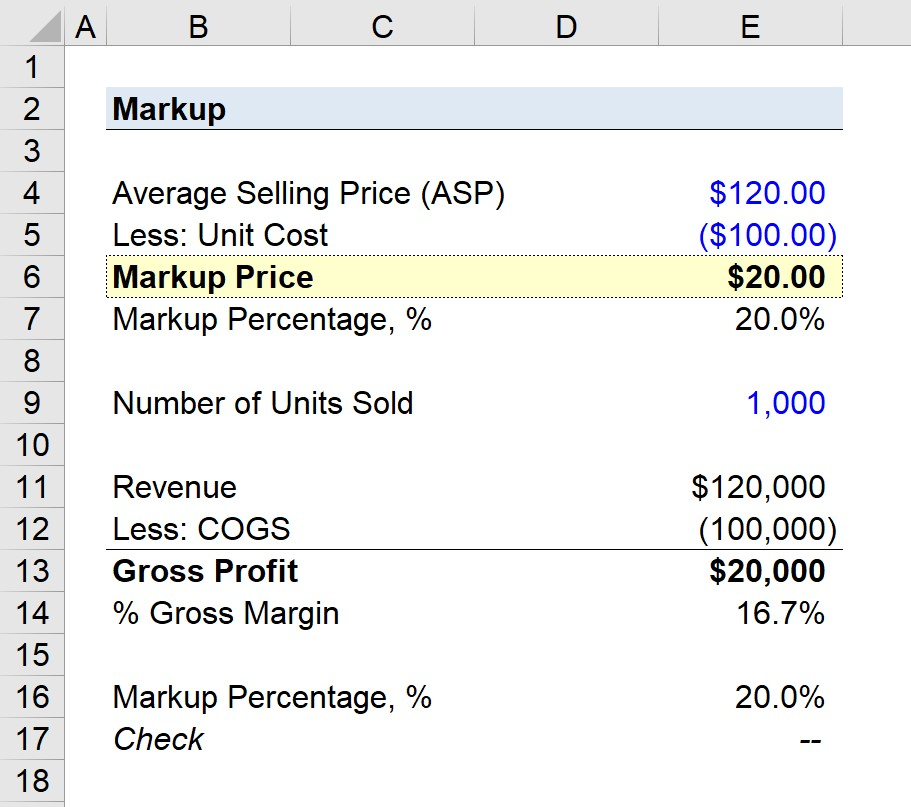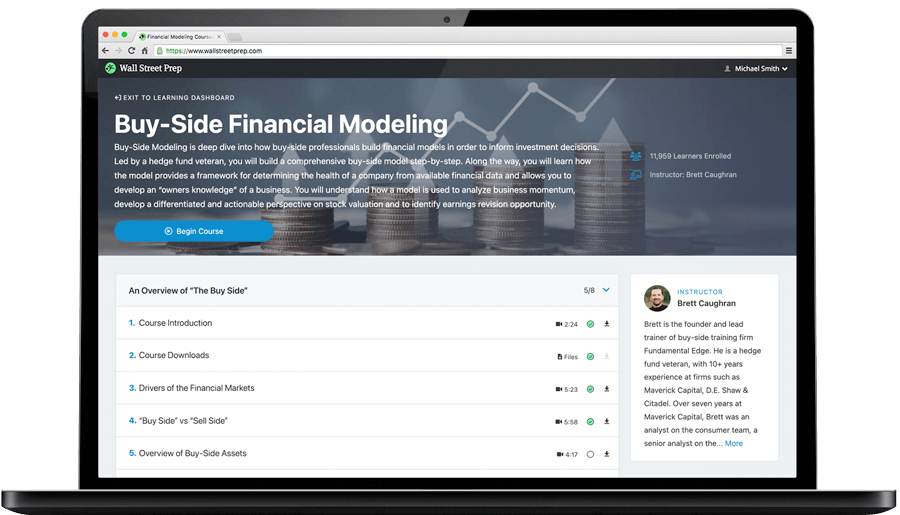Welcome to Wall Street Prep! Use code at checkout for 15% off.# Markup

Guide to Understanding the Markup Concept## How to Calculate the Markup?

The markup price represents the average selling price (ASP) in excess of the cost of production per unit.

• Average Selling Price (ASP) → The simplest approach to calculating a company’s ASP is to divide a company’s revenue by the total number of units sold, but if the product line consists of a broad range of products with large variances in pricing (and volume), the recommended approach is to calculate the ASP on a per product category basis.
• Cost Per Unit → The cost per unit is the cost of production on a per-unit basis, and the metric is inclusive of any costs associated with the production process (i.e. sum of all production costs divided by the number of units sold).

Calculating the markup is a rather straightforward process, as it simply involves:

1. Estimating the Average Selling Price (ASP)
2. Subtracting the Average Unit Cost from the ASP

## Markup Formula

The formula for calculating the markup price is as follows.

###### Formula
• Markup = Average Selling Price Per Unit – Average Unit Cost

In order to make the markup price metric more practical, the markup can be divided by the unit cost to arrive at the markup percentage.

The markup percentage is the excess ASP per unit (i.e. the markup price) divided by the unit cost.

###### Formula
• Markup Percentage = Markup Price / Average Unit Cost

Since all companies seek to improve their operating efficiency and profit margins over time, management must set prices accordingly to ensure they are on track to become more profitable.

## Mark-Up vs. Profit Margin: What is the Difference?

The mark-up and profit margins of a particular company are closely tied concepts.

The higher the mark-up, the higher the margin profile of the company – all else being equal.

While a company’s margins divide a specific profit metric by revenue, a markup reflects how much more the selling price is than the cost of production.

For instance, the gross profit margin divides a company’s gross profit by revenue, which equals revenue less the cost of goods sold (COGS). The gross margin portrays the percentage of revenue remaining after COGS are deducted.

The relationship between the mark-up and gross margin is that the mark-up percentage can be back-solved by dividing the gross margin by COGS.

###### Gross Margin to Mark-Up Percentage Formula
• Mark-Up Percentage = Gross Margin / COGS

If COGS was entered as a negative figure in Excel, make sure to place a negative sign in front of the formula.

## Markup Calculator – Excel Template

We’ll now move to a modeling exercise, which you can access by filling out the form below.Submitting...

## Markup Calculation Example

Suppose a company’s products are sold at an average selling price of \$120, while the associated unit cost is \$100.

• Average Selling Price (ASP) = \$120.00
• Unit Cost = \$100.00

By subtracting the unit cost from the average selling price (ASP), we arrive at a markup price of \$20, i.e. the excess ASP over the unit cost of production.

• Markup = \$120.00 – \$100.00 = \$20.00

By dividing the \$20 markup by the \$100 unit cost, the implied markup percentage is 20%.

• Markup Percentage = \$20 / \$100 = 0.20, or 20%

Next, we’ll assume that our hypothetical company sold 1,000 units of its product in a specified period.

The revenue for the period is \$120k while COGS is \$100k, which we calculated by multiplying the ASP by the number of units sold, and the unit cost by the number of units sold, respectively.

• Revenue = \$120,000
• COGS = \$100,000
• Gross Profit = \$120,000 – \$100,000 = \$20,000

The gross profit is \$20k, and we’ll divide that amount by the \$120k in revenue to calculate the gross margin as 16.7%.

In closing, the \$20k in gross profit can be divided by the \$100k in COGS to confirm the markup percentage is 20%.Step-by-Step Online Course

### Learn Buy-Side (Hedge Fund) Modeling

Led by a former hedge fund PM (Maverick, Citadel, DE Shaw, Schonfeld), this program begins where financial modeling training ends — with a deep-dive into how buy-side analysts build financial models to make key investment decisions.

Inline Feedbacks